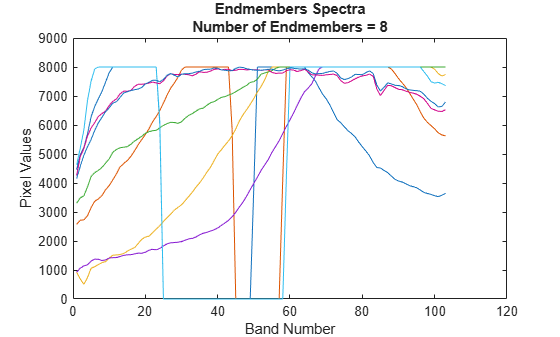# fippi

Extract endmember signatures using fast iterative pixel purity index

## Syntax

``endmembers = fippi(inputData,numEndmembers)``
``endmembers = fippi(inputData,numEndmembers,'ReductionMethod',method)``

## Description

example

````endmembers = fippi(inputData,numEndmembers)` extracts endmember signatures from hyperspectral data `inputData` by using the fast iterative pixel purity index (FIPPI) algorithm. `numEndmembers` is the number of endmember signatures to be extracted using the FIPPI algorithm. For more information about the FIPPI method, see Algorithms.```

example

````endmembers = fippi(inputData,numEndmembers,'ReductionMethod',method)` additionally specifies the option for selecting the dimensionality reduction method to be used before computing the endmembers. NoteThis function requires the Image Processing Toolbox™ Hyperspectral Imaging Library. You can install the Image Processing Toolbox Hyperspectral Imaging Library from Add-On Explorer. For more information about installing add-ons, see Get and Manage Add-Ons. ```

## Examples

collapse all

Read a hyperspectral data into the workspace.

`hcube = hypercube('paviaU.hdr');`

Find the number of spectrally distinct endmembers present in the hyperspectral data cube by using `countEndmembersHFC` function.

`numEndmembers = countEndmembersHFC(hcube,'PFA',10^-7);`

Compute the endmembers using the fast iterative pixel purity index (FIPPI) method. By default, the `fippi` function uses maximum noise fraction (MNF) transform for preprocessing.

`endmembers = fippi(hcube.DataCube,numEndmembers);`

Plot the endmembers of the hyperspectral data.

```figure plot(endmembers) xlabel('Band Number') ylabel('Pixel Values') ylim([0 9000]) title({'Endmembers Spectra',['Number of Endmembers = ' num2str(numEndmembers)]});```Read a hyperspectral data into the workspace.

`hcube = hypercube('paviaU.hdr');`

Find the number of spectrally distinct endmembers present in the hyperspectral data cube by using `countEndmembersHFC` function.

`numEndmembers = countEndmembersHFC(hcube,'PFA',10^-7);`

Compute the endmembers using the fast iterative pixel purity index (FIPPI) method. Select principal component analysis (PCA) as the dimensionality reduction method for preprocessing.

`endmembers = fippi(hcube.DataCube,numEndmembers,'ReductionMethod','PCA');`

Plot the endmembers of the hyperspectral data.

```figure plot(endmembers) xlabel('Band Number') ylabel('Pixel Values') ylim([0 9000]) title({'Endmembers Spectra',['Number of Endmembers = ' num2str(numEndmembers)]});```## Input Arguments

collapse all

Input hyperspectral data, specified as an 3-D numeric array or a `hypercube` object. If the input is a `hypercube` object, then the function reads the hyperspectral data from its `DataCube` property.

The hyperspectral data is an numeric array of size M-by-N-by-C. M andN are the number of rows and columns in the hyperspectral data respectively. C is the number of spectral bands in the hyperspectral data.

Data Types: `single` | `double` | `int8` | `int16` | `int32` | `int64` | `uint8` | `uint16` | `uint32` | `uint64`

Number of endmembers to be extracted, specified as a positive scalar integer. The value must be in the range [`1` C]. C is the number of spectral bands in the input hyperspectral data. You can find the number of spectrally distinct endmembers in the input data by using the `countEndmembersHFC` function.

Data Types: `single` | `double` | `int8` | `int16` | `int32` | `int64` | `uint8` | `uint16` | `uint32` | `uint64`

Dimensionality reduction method, specified as a comma-separated pair of '`ReductionMethod`' and one of `'MNF'` or `'PCA'`.

Specify the value as

• `'MNF'` — To perform dimensionality reduction using the maximum noise fraction (MNF) method.

• `'PCA'` — To perform dimensionality reduction using the principal component analysis (PCA) method.

The function computes the endmembers from the reduced data.

Data Types: `char` | `string`

## Output Arguments

collapse all

Endmember signatures, returned as a matrix of size C-by-K and datatype same as the input hyperspectral data.

Data Types: `single` | `double` | `int8` | `int16` | `int32` | `int64` | `uint8` | `uint16` | `uint32` | `uint64`

## Algorithms

FIPPI is an iterative approach that iteratively selects the better candidates for endmembers after each iteration. Unlike pixel purity index (PPI) technique, the FIPPI method selects the initial set of skewers by using the automatic target generation process (ATGP) . As a result the algorithm converges faster and generates unique pixel for each endmember. The steps involved in FIPPI approach are summarized as follows:

1. Compute principal component bands and reduce the spectral dimensionality of the input data by using MNF or PCA. The number of principal component bands to be extracted is set equal to the number of endmembers to be extracted.

2. Find the initial set of endmembers by using the ATGP method. The initial set of endmembers form the set of skewers ${\left\{{\text{skewer}}_{j}^{\left(1\right)}\right\}}_{j\text{\hspace{0.17em}}=\text{\hspace{0.17em}}1}^{p}$ onto which you project the input data.

3. For iteration 1, Let r1 be a sample vector that denote a pixel spectra. Then, orthogonally project the sample vector onto each skewers and find the extrema.

4. Store the location of each extreme value and count their occurrences. The number of occurrences is known as the PPI count.

5. Find the PPI count for each pixel spectra and identify the set of sample vectors {rk} with maximum PPI count as endmembers.

6. Generate a new set of skewers by combining the set of new endmembers with the initial set of skewers.

`$\left\{{\text{skewer}}_{j}^{\left(2\right)}\right\}\text{\hspace{0.17em}}=\text{\hspace{0.17em}}\text{\hspace{0.17em}}\left\{{\text{r}}_{k}^{\left(1\right)}\right\}\cup \left\{{\text{skewer}}_{j}^{\left(1\right)}\right\}$`

7. For iteration 2, project all the sample vectors onto the new set of skewers and identify the new set of endmembers. Then, generate the new set of skewers for the next iteration, $\left\{{\text{skewer}}_{j}^{\left(3\right)}\right\}\text{\hspace{0.17em}}$.

8. The iteration stops, if the set of skewers generated in two consecutive iterations remain same. This final set of skewers are the endmembers of the input data.

`$\left\{{\text{skewer}}_{j}^{\left(n+1\right)}\right\}\text{\hspace{0.17em}}=\text{\hspace{0.17em}}\text{\hspace{0.17em}}\left\{{\text{skewer}}_{j}^{\left(n\right)}\right\}\text{\hspace{0.17em}}$`

 Chang, C.-I., and A. Plaza. “A Fast Iterative Algorithm for Implementation of Pixel Purity Index.” IEEE Geoscience and Remote Sensing Letters 3, no. 1 (January 2006): 63–67. https://doi.org/10.1109/LGRS.2005.856701.